## RD Sharma Class 8 Solutions Chapter 18 Practical Geometry Ex 18.1

These Solutions are part of RD Sharma Class 8 Solutions. Here we have given RD Sharma Class 8 Solutions Chapter 18 Practical Geometry Ex 18.1

Other Exercises

Question 1.
Construct a quadrilateral ABCD in which AB = 4.4 cm, BC = 4 cm, CD = 6.4 cm, DA = 3.8 cm and BD = 6.6 cm.
Solution:
Steps of construction :
(i) Draw a line segment AB = 4.4 cm.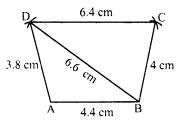(ii) With centre A and radius 3.8 cm and with centre B and radius 6.6 cm, draw arcs intersecting each other at D.
(iii) With centre B and radius 4 cm, and with centre D and radius 6.4 cm, draw arcs intersecting each other at C on the other side of BD.
(iv) Join AD, BD, BC and DC.
The ABCD is the required quadrilateral.

Question 2.
Construct a quadrilateral ABCD such that AB = BC = 5.5 cm, CD = 4 cm, DA = 6.3 cm and AC = 9.4 cm. Measure BD.
Solution:
(i) Draw a line segment AC = 9.4 cm.
(ii) With centre A and C and radius 5.5 cm, draw arcs intersecting each other at B.
(iii) Join AB and CB.
(iv) Again with centre A and radius 6.3 cm, and with centre C and radius 4 cm, draw arcs intersecting each other at D below the line segment AC.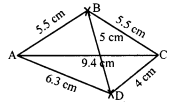Then ABCD is the required quadrilateral. On measuring BD, it is 5 cm.

Question 3.
Construct a quadrilateral XYZW in which XY = 5 cm, YZ = 6 cm, ZW = 7 cm, WX = 3 cm and XZ = 9 cm.
Solution:
Steps of construction :
(i) Draw a line segment XZ = 9 cm.
(ii) With centre X and radius 3 cm and with centre Z and radius 7 cm, draw arcs intersecting each other at W.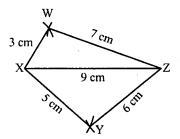(iii) Join XW and ZW.
(iv) Again with centre X and radius 5 cm and with centre Z and radius 6 cm, draw arcs, intersecting each other at Y below the line segment XZ.
(v) Join XY and ZY.
Then XYZW is the required quadrilateral.

Question 4.
Construct a parallelogram PQRS such that PQ = 5.2 cm, PR = 6.8 cm and QS = 8.2 cm.
Solution:
Steps of construction:
In a parallelogram, diagonals bisect each other. Now
(i) Draw a line segment PQ = 5.2 cm.
(ii) With centre P and radius 3.4 cm ($$\frac { 1 }{ 2 }$$ of PR) and with centre Q and radius 4.1 cm ($$\frac { 1 }{ 2 }$$ of QS) draw arcs intersecting each other at O.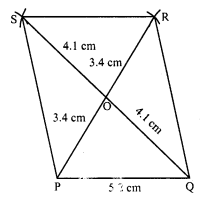(iii) Join PQ and QO and produced them to R and S respectively such that PO = OR and QO = OS.
(iv) Join PS, SR and RQ.
Then PQRS is the required parallelogram.

Question 5.
Construct a rhombus with side 6 cm and one diagonal 8 cm. Measure the other diagonal.
Solution:
Steps of construction :
Sides of a rhombus are equal.
(i) Draw a line segment AC = 8 cm.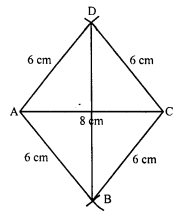(ii) With centres A and C and radius 6 cm, draw two arcs above the line segment AC and two below the line segment AC, intersecting each other at D and B respectively.
(iii) Join AB, AD, BC and CD.
Then ABCD is the required rhombus.
JoinBD.
On measuring BD, it is approximately 9 cm

Question 6.
Construct a kite ABCD in which AB = 4 cm, BC = 4.9 cm and AC = 7.2 cm.
Solution:
Steps of construction :
(i) Draw a line segment AC = 7.2 cm.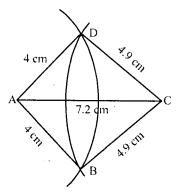(ii) With centre A and radius 4 cm draw an arc.
(iii) With centre C and radius 4.9 cm, draw another arc which intersects the first arc at B and D.
(iv) Join AB, BC, CD and DA.
Then ABCD is the required kite.

Question 7.
Construct, if possible, a quadrilateral ABCD given, AB = 6 cm BC = 3.7 cm, CD = 5.7 cm, AD = 5.5 cm and BD = 6.1 cm. Give reasons for not being able to construct, if you cannot.
Solution:
Steps of construction :
(i) Draw a line segment BD = 6.1 cm.
(ii) With centre B and radius 6 cm and with centre D and radius 5.5 cm, draw arcs intersecting at A.
(iv) Again with centre B and radius 3.7 cm and with centre D and radius 5.7 cm, draw two arcs intersecting each other at C below the BD.(v) Join BC and DC.
Then ABCD is the required quadrilateral.

Question 8.
Construct, if possible a quadrilateral ABCD in which AB = 6 cm, BC = 7 cm, CD = 3 cm, AD = 5.5. cm and AC = 11 cm. Give reasons for not being able to construct, if you cannot.
Solution:
Steps of construction:
It is not possible to construct this quadrilateral ABCD because
AD + DC = 5.5 cm + 3 cm = 8.5 cm
and AC = 11 cm